Related Articles

# Python | pandas.to_numeric method

• Last Updated : 17 Dec, 2018

Python is a great language for doing data analysis, primarily because of the fantastic ecosystem of data-centric python packages. Pandas is one of those packages and makes importing and analyzing data much easier.

`pandas.to_numeric()` is one of the general functions in Pandas which is used to convert argument to a numeric type.

Attention geek! Strengthen your foundations with the Python Programming Foundation Course and learn the basics.

To begin with, your interview preparations Enhance your Data Structures concepts with the Python DS Course. And to begin with your Machine Learning Journey, join the Machine Learning - Basic Level Course

Syntax: pandas.to_numeric(arg, errors=’raise’, downcast=None)

Parameters:
arg : list, tuple, 1-d array, or Series
errors : {‘ignore’, ‘raise’, ‘coerce’}, default ‘raise’
-> If ‘raise’, then invalid parsing will raise an exception
-> If ‘coerce’, then invalid parsing will be set as NaN
-> If ‘ignore’, then invalid parsing will return the input
downcast : [default None] If not None, and if the data has been successfully cast to a numerical dtype downcast that resulting data to the smallest numerical dtype possible according to the following rules:
-> ‘integer’ or ‘signed’: smallest signed int dtype (min.: np.int8)
-> ‘unsigned’: smallest unsigned int dtype (min.: np.uint8)
-> ‘float’: smallest float dtype (min.: np.float32)

Returns: numeric if parsing succeeded. Note that return type depends on input. Series if Series, otherwise ndarray.

Code #1:

Observe this dataset first. We’ll use ‘Numbers’ column of this data in order to make Series and then do the operation.

 `# importing pandas module ``import` `pandas as pd ``   ` `# making data frame ``df ``=` `pd.read_csv(``"https://media.geeksforgeeks.org/wp-content/uploads/nba.csv"``) `` ` `df.head(``10``)`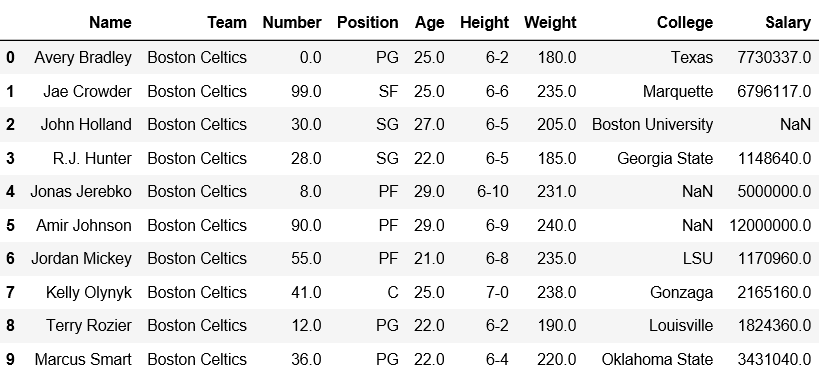Calling Series constructor on Number column and then selecting first 10 rows.

 `# importing pandas module ``import` `pandas as pd ``   ` `# making data frame ``df ``=` `pd.read_csv(``"nba.csv"``) `` ` ` ` `# get first ten 'numbers'``ser ``=` `pd.Series(df[``'Number'``]).head(``10``)``ser`

Output: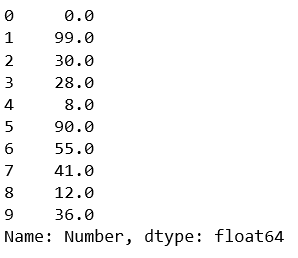Using pd.to_numeric() method. Observe that by using downcast=’signed’, all the values will be casted to integer.

 `pd.to_numeric(ser, downcast ``=``'signed'``)`

Output: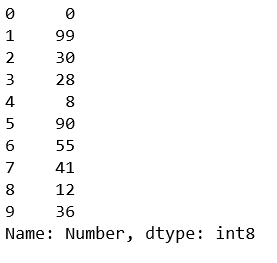Code #2: Using errors=’ignore’. It will ignore all non-numeric values.

 `# importing pandas module ``import` `pandas as pd ``   ` ` ` `# get first ten 'numbers'``ser ``=` `pd.Series([``'Geeks'``, ``11``, ``22.7``, ``33``])`` ` `pd.to_numeric(ser, errors ``=``'ignore'``)`

Output: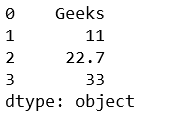Code #3: Using errors=’coerce’. It will replace all non-numeric values with NaN.

 `# importing pandas module ``import` `pandas as pd ``   ` ` ` `# get first ten 'numbers'``ser ``=` `pd.Series([``'Geeks'``, ``11``, ``22.7``, ``33``])`` ` `pd.to_numeric(ser, errors ``=``'coerce'``)`

Output: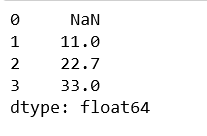My Personal Notes arrow_drop_up